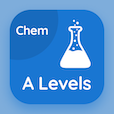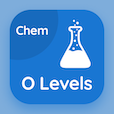Engineering Online Courses

Engineering Physics MCQs

Engineering Physics MCQ PDF - Topics

# Density of Selected Materials of Engineering Interest MCQ Quiz Online

Learn Density of Selected Materials of Engineering Interest Multiple Choice Questions (MCQ), Density of Selected Materials of Engineering Interest quiz answers PDF to study engineering physics online course for engineering physics classes. Equilibrium, Indeterminate Structures Multiple Choice Questions and Answers (MCQs), Density of Selected Materials of Engineering Interest quiz questions for tricky trivia questions. "Density of Selected Materials of Engineering Interest MCQ" PDF Book: center of gravity, density of selected materials of engineering interest, ultimate and yield strength of selected materials of engineering interest test prep for online colleges enrolling.

"Aluminium has a density of" MCQ PDF: density of selected materials of engineering interest with choices 2320 kg/m3, 7860 kg/m3, 2700 kg/m3, and 2190 kg/m3 for tricky trivia questions. Study density of selected materials of engineering interest quiz questions for merit scholarship test and certificate programs for college entrance exams.

## MCQs on Density of Selected Materials of Engineering Interest Quiz

MCQ: Aluminium has a density of

2320 kg/m3
7860 kg/m3
2700 kg/m3
2190 kg/m3

MCQ: 1050 kg/m3 is a density of

polystyrene
bone
wood
glass

MCQ: Bone has a density of

525 kg/m3
1900 kg/m3
860 kg/m3
1050 kg/m3

MCQ: Steel has a density of

7860 kg/m3
2710 kg/m3
2190 kg/m3
2320 kg/m3

MCQ: Douglas fir wood has a density of

525 kg/m3
860 kg/m3
1900 kg/m3
1050 kg/m3

### More Topics from Engineering Physics Course